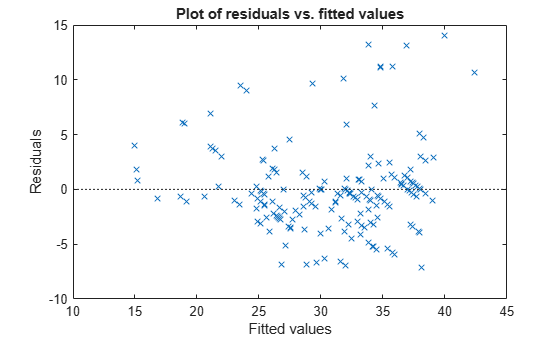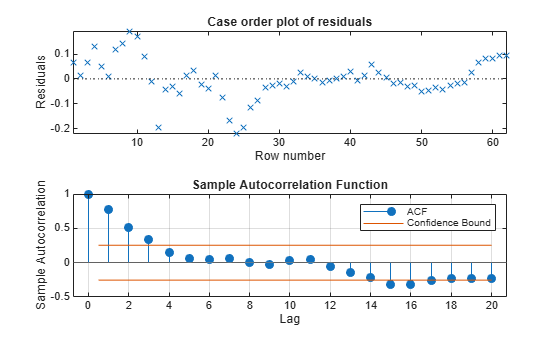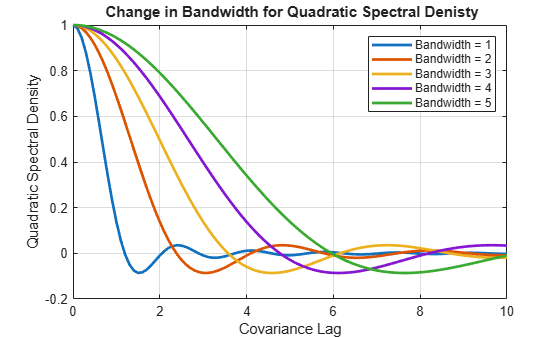# hac

Heteroscedasticity and autocorrelation consistent covariance estimators

## Syntax

EstCov = hac(X,y)
EstCov = hac(Tbl)
EstCov = hac(Mdl)
EstCov = hac(___,Name,Value)
[EstCov,se,coeff] = hac(___)

## Description

example

EstCov = hac(X,y) returns robust covariance estimates for ordinary least squares (OLS) coefficient estimates of multiple linear regression models y = Xβ + ε under general forms of heteroscedasticity and autocorrelation in the innovations process ε.NaNs in the data indicate missing values, which hac removes using list-wise deletion. hac sets Data = [X y], then it removes any row in Data containing at least one NaN. This reduces the effective sample size, and changes the time base of the series.

example

EstCov = hac(Tbl) returns robust covariance estimates for OLS coefficient estimates of multiple linear regression models, with predictor data, X, in the first numPreds columns of the tabular array, Tbl, and response data, y, in the last column.hac removes all missing values in Tbl, indicated by NaNs, using list-wise deletion. In other words, hac removes all rows in Tbl containing at least one NaN. This reduces the effective sample size, and changes the time base of the series.

example

EstCov = hac(Mdl) returns robust covariance estimates for OLS coefficient estimates from a fitted multiple linear regression model, Mdl, as returned by fitlm.

example

EstCov = hac(___,Name,Value) uses any of the input arguments in the previous syntaxes and additional options that you specify by one or more Name,Value pair arguments.For example, use Name,Value pair arguments to choose weights for HAC or HC estimators, set a bandwidth for a HAC estimator, or prewhiten the residuals.

example

[EstCov,se,coeff] = hac(___) additionally returns a vector of corrected coefficient standard errors, se = sqrt(diag(EstCov)), and a vector of OLS coefficient estimates, coeff.

## Examples

collapse all

Model an automobile's price with its curb weight, engine size, and cylinder bore diameter using the linear model:

${\text{price}}_{i}={\beta }_{0}+{\beta }_{1}{\text{curbWeight}}_{i}+{\beta }_{2}{\text{engineSize}}_{i}+{\beta }_{3}{\text{bore}}_{i}+{\epsilon }_{i}.$

Estimate model coefficients and White's robust covariance.

Load the 1985 automobile imports data set (Frank and Asuncion, 2012). Extract the columns that correspond to the predictor and response variables.

load imports-85 Tbl = table(X(:,7),X(:,8),X(:,9),X(:,15),... 'Variablenames',{'curbWeight','engineSize',... 'bore','price'});

Fit the linear model to the data and plot the residuals versus the fitted values.

Mdl = fitlm(Tbl); plotResiduals(Mdl,'fitted')The residuals seem to flare out, which indicates heteroscedasticity.

Compare the coefficient covariance estimate from OLS and from using hac to calculate White's heteroscedasticity robust estimate.

[LSCov,LSSe,coeff] = hac(Mdl,'type','HC','weights',... 'CLM','display','off'); %Usual OLS estimates, also found in %Mdl.CoefficientCovariance LSCov
LSCov = 4×4 13.7124 0.0000 0.0120 -4.5609 0.0000 0.0000 -0.0000 -0.0005 0.0120 -0.0000 0.0002 -0.0017 -4.5609 -0.0005 -0.0017 1.8195 
[WhiteCov,WhiteSe,coeff] = hac(Mdl,'type','HC','weights',... 'HC0','display','off'); % White's estimates WhiteCov
WhiteCov = 4×4 15.5122 -0.0008 0.0137 -4.4461 -0.0008 0.0000 -0.0000 -0.0003 0.0137 -0.0000 0.0001 -0.0010 -4.4461 -0.0003 -0.0010 1.5707 

The OLS coefficient covariance estimate is not equal to White's robust estimate because the latter accounts for the heteroscedasticity in the residuals.

Model nominal GNP (GNPN) with consumer price index (CPI), real wages (WR), and the money stock (MS) using the linear model:

${\text{GNPN}}_{i}={\beta }_{0}+{\beta }_{1}{\text{CPI}}_{i}+{\beta }_{2}{\text{WR}}_{i}+{\beta }_{3}{\text{MS}}_{i}+{\epsilon }_{i}.$

Estimate the model coefficients and the Newey-West OLS coefficient covariance matrix.

Load the Nelson Plosser data set.

load Data_NelsonPlosser Tbl = DataTable(:,[8,10,11,2]); % Tabular array containing the variables T = sum(~any(ismissing(Tbl),2)); % Remove NaNs to obtain sample size y = Tbl{:,4}; % Numeric response X = Tbl{:,1:3}; % Numeric matrix of predictors

Fit the linear model. Remove the beginning block of NaN values in the residual vector for autocorr.

Mdl = fitlm(X,y); resid = Mdl.Residuals.Raw(~isnan(Mdl.Residuals.Raw)); figure subplot(2,1,1) hold on plotResiduals(Mdl,'fitted') axis tight plot([min(Mdl.Fitted) max(Mdl.Fitted)],[0 0],'k-') title('Residual Plot') xlabel('$\hat y$','Interpreter','latex') ylabel('Residuals') axis tight subplot(2,1,2) autocorr(resid)The residual plot exhibits signs of heteroscedasticity, autocorrelation, and possibly model misspecification. The sample autocorrelation function clearly exhibits autocorrelation.

Calculate the lag selection parameter for the standard Newey-West HAC estimate (Andrews and Monohan, 1992).

maxLag = floor(4*(T/100)^(2/9));

Estimate the standard Newey-West OLS coefficient covariance using hac by setting the bandwidth to maxLag + 1. Display the OLS coefficient estimates, their standard errors, and the covariance matrix.

EstCov = hac(X,y,'bandwidth',maxLag+1,'display','full');
Estimator type: HAC Estimation method: BT Bandwidth: 4.0000 Whitening order: 0 Effective sample size: 62 Small sample correction: on Coefficient Estimates: | Coeff SE -------------------------- Const | 20.2317 35.0767 x1 | -0.1000 0.7965 x2 | -1.5602 1.1546 x3 | 2.6329 0.2043 Coefficient Covariances: | Const x1 x2 x3 ------------------------------------------------ Const | 1230.3727 -15.3285 -24.2677 6.7855 x1 | -15.3285 0.6343 -0.2960 -0.0957 x2 | -24.2677 -0.2960 1.3331 -0.1285 x3 | 6.7855 -0.0957 -0.1285 0.0418 

The first column in the output contains the OLS estimates (${\underset{}{\overset{ˆ}{\beta }}}_{0},...,{\underset{}{\overset{ˆ}{\beta }}}_{3}$, respectively), and the second column contains their standard errors. The last four columns contained in the table represent the estimated coefficient covariance matrix. For example, $Cov\left({\underset{}{\overset{ˆ}{\beta }}}_{1},{\underset{}{\overset{ˆ}{\beta }}}_{2}\right)=-0.2960$.

Alternatively, pass in a tabular array to hac.

EstCov = hac(Tbl,'bandwidth',maxLag+1,'display','off');

The advantage of passing in a tabular array is that the top and left margins of the covariance table use the variable names.

Plot the kernel density functions available in hac.

Set domain, x, and range w.

x = (0:0.001:3.2)'; w = zeros(size(x));

Compute the truncated kernel density.

cTR = 2; % Renormalization constant TR = (abs(x) <= 1); TRRn = (abs(cTR*x) <= 1); wTR = w; wTR(TR) = 1; wTRRn = w; wTRRn(TRRn) = 1;

Compute the Bartlett kernel density.

cBT = 2/3; % Renormalization constant BT = (abs(x) <= 1); BTRn = (abs(cBT*x) <= 1); wBT = w; wBT(BT) = 1-abs(x(BT)); wBTRn = w; wBTRn(BTRn) = 1-abs(cBT*x(BTRn));

Compute the Parzen kernel density.

cPZ = 0.539285; % Renormalization constant PZ1 = (abs(x) >= 0) & (abs(x) <= 1/2); PZ2 = (abs(x) >= 1/2) & (abs(x) <= 1); PZ1Rn = (abs(cPZ*x) >= 0) & (abs(cPZ*x) <= 1/2); PZ2Rn = (abs(cPZ*x) >= 1/2) & (abs(cPZ*x) <= 1); wPZ = w; wPZ(PZ1) = 1-6*x(PZ1).^2+6*abs(x(PZ1)).^3; wPZ(PZ2) = 2*(1-abs(x(PZ2))).^3; wPZRn = w; wPZRn(PZ1Rn) = 1-6*(cPZ*x(PZ1Rn)).^2 ... + 6*abs(cPZ*x(PZ1Rn)).^3; wPZRn(PZ2Rn) = 2*(1-abs(cPZ*x(PZ2Rn))).^3;

Compute the Tukey-Hanning kernel density.

cTH = 3/4; % Renormalization constant TH = (abs(x) <= 1); THRn = (abs(cTH*x) <= 1); wTH = w; wTH(TH) = (1+cos(pi*x(TH)))/2; wTHRn = w; wTHRn(THRn) = (1+cos(pi*cTH*x(THRn)))/2;

Compute the quadratic spectral kernel density.

argQS = 6*pi*x/5; w1 = 3./(argQS.^2); w2 = (sin(argQS)./argQS)-cos(argQS); wQS = w1.*w2; wQS(x == 0) = 1; wQSRn = wQS; % Renormalization constant = 1

Plot the kernel densities.

figure plot(x,[wTR,wBT,wPZ,wTH,wQS],'LineWidth',2) hold on plot(x,w,'k','LineWidth',2) axis([0 3.2 -0.2 1.2]) grid on title('{\bf HAC Kernels}') legend({'Truncated','Bartlett','Parzen','Tukey-Hanning',... 'Quadratic Spectral'}) xlabel('Covariance Lag') ylabel('Weight')All graphs are truncated at Covariance Lag = 1, except for the quadratic spectral. The quadratic spectral density approaches 0 as Covariance Lag gets large, but does not get truncated.

Plot renormalized kernels. Unlike the densities in the previous plot, these have the same asymptotic variance (Andrews, 1991).

figure plot(x,[wTRRn,wBTRn,wPZRn,wTHRn,wQSRn],'LineWidth',2) hold on plot(x,w,'k','LineWidth',2) axis([0 3.2 -0.2 1.2]) grid on title('{\bf Renormalized HAC Kernels} (Equal Asymptotic Variance)') legend({'Truncated','Bartlett','Parzen','Tukey-Hanning',... 'Quadratic Spectral'}) xlabel('Covariance Lag') ylabel('Weight')Examine the effects of changing the bandwidth parameter on the quadratic spectral density.

Assign several bandwidth values to b. Assign the domain to l. Calculate x = l/|b|.

b = (1:5)'; l = (0:0.1:10); x = bsxfun(@rdivide,repmat(l,[size(b),1]),b)';

Calculate the quadratic spectral density under the domain for each bandwidth value.

argQS = 6*pi*x/5; w1 = 3./(argQS.^2); w2 = (sin(argQS)./argQS)-cos(argQS); wQS = w1.*w2; wQS(x == 0) = 1;

figure; plot(l,wQS,'LineWidth',2); grid on; xlabel('Covariance Lag'); ylabel('Quadratic Spectral Density'); title('Change in Bandwidth for Quadratic Spectral Denisty'); legend('Bandwidth = 1','Bandwidth = 2','Bandwidth = 3',... 'Bandwidth = 4','Bandwidth = 5');As the bandwidth increases, the kernel imparts more weight to larger lags.

## Input Arguments

collapse all

Predictor data for the multiple linear regression model, specified as a numObs-by-numPreds numeric matrix.

numObs is the number of observations and numPreds is the number of predictor variables.

Data Types: double

Response data for the multiple linear regression model, specified as a numObs-by-1 vector with numeric or logical entries.

Data Types: double | logical

Predictor and response data for the multiple linear regression model, specified as a numObs-by-numPreds + 1 tabular array.

The first numPreds variables of Tbl are the predictor data, and the last variable is the response data.

The predictor data must be numeric, and the response data must be numeric or logical.

Data Types: table

Fitted linear model, specified as a model returned by fitlm.

### Name-Value Pair Arguments

Specify optional comma-separated pairs of Name,Value arguments. Name is the argument name and Value is the corresponding value. Name must appear inside quotes. You can specify several name and value pair arguments in any order as Name1,Value1,...,NameN,ValueN.

Example: 'type','HAC','bandwidth',floor(4*(T/100)^(2/9))+1,'weights','BT' specifies the standard Newey-West OLS coefficient covariance estimate.

Variable names used in displays and plots of the results, specified as the comma-separated pair consisting of 'varNames' and a string vector or cell vector of character vectors. varNames must have length numPreds, and each cell corresponds to a variable name. The software truncates all variable names to the first five characters.

varNames must include variable names for all variables in the model, such as an intercept term (e.g., 'Const') or higher-order terms (e.g., 'x1^2' or 'x1:x2').

The default variable names for:

• The matrix X is the cell vector of character vectors {'x1','x2',...}

• The tabular array Tbl is the property Tbl.Properties.VariableNames

• The linear model Mdl is the property Mdl.CoefficientNames

Example: 'varNames',{'Const','AGE','BBD'}

Data Types: cell | string

Indicate whether to include model intercept when hac fits the model, specified as the comma-separated pair consisting of 'intercept' and a logical value.

ValueDescription
trueInclude an intercept in the model.
falseExclude an intercept from the model.

If you specify Mdl, then hac ignores intercept and uses the intercept in Mdl.

Example: 'intercept',false

Data Types: logical

Coefficient covariance estimator type, specified as the comma-separated pair consisting of 'type' and a value in this table.

ValueCovariance EstimateUsage
'HAC'Return heteroscedasticity-and-autocorrelation-consistent (HAC) estimate as described in , , , and .When residuals exhibit both heteroscedasticity and autocorrelation
'HC'Return heteroscedasticity-consistent (HC) estimate as described in , , and .When residuals exhibit only heteroscedasticity

Example: 'type','HC'

Coefficient covariance estimator weighting scheme, specified as the comma-specified pair consisting of 'weights' and a string vector, character vector, or length numObs numeric vector.

Set 'weights' to specify the structure of the innovations covariance Ω. hac uses this specification to compute $\stackrel{^}{\Phi }={X}^{\prime }\stackrel{^}{\Omega }X$ (see Sandwich Estimators).

• If type is HC, then $\stackrel{^}{\Omega }=diag\left(\omega \right),$ where ωi estimates the ith innovation variance, i = 1,...,T, and T = numObs. hac estimates ωi using the ith residual, εi, its leverage ${h}_{i}={x}_{i}{\left({X}^{\prime }X\right)}^{-1}{x}_{i}^{\prime },$ ${d}_{i}=\mathrm{min}\left(4,\frac{{h}_{i}}{\overline{h}}\right),$ and the degrees of freedom, dfe.

Use the following table to choose 'weights'.

ValueWeightReference
'CLM'

${\omega }_{i}=\frac{1}{dfe}\sum _{i=1}^{T}{\epsilon }_{i}^{2}$


'HCO' (default when 'type','HC')

${\omega }_{i}={\epsilon }_{i}^{2}$


'HC1'

${\omega }_{i}=\frac{T}{dfe}{\epsilon }_{i}^{2}$


'HC2'

${\omega }_{i}=\frac{{\epsilon }_{i}^{2}}{1-{h}_{i}}$


'HC3'

${\omega }_{i}=\frac{{\epsilon }_{i}^{2}}{{\left(1-{h}_{i}\right)}^{2}}$


'HC4'

${\omega }_{i}=\frac{{\epsilon }_{i}^{2}}{{\left(1-{h}_{i}\right)}^{{d}_{i}}}$



• If type is HAC, then hac weights the component products that form $\stackrel{^}{\Phi }$, ${x}_{i}{}^{\prime }{\epsilon }_{i}{\epsilon }_{j}{x}_{j}$, using a measure of autocorrelation strength, ω(l), at each lag, l = |ij|. ω(l) = k(l/b), where k is a kernel density estimator and b is a bandwidth specified by 'bandwidth'.

Use the following table to choose 'weights'.

ValueKernel DensityKernel Density FunctionReference
'TR'

Truncated


'BT' (default when 'type','HAC')

Bartlett


'PZ'

Parzen


'TH'

Tukey-Hanning


'QS'

$k\left(z\right)=\frac{25}{12{\pi }^{2}{z}^{2}}\left(\frac{\mathrm{sin}\left(6\pi z/5\right)}{6\pi z/5}-\mathrm{cos}\left(6\pi z/5\right)\right)$



For a visual description of these kernel densities, see Plot Kernel Densities.

• For either type, you can set 'weights' to any length numObs numeric vector without containing NaNs. However, a user-defined weights vector might not produce positive definite matrices.

If you set weights to a numeric vector, then hac sets Data = [X y weights] = [DS weights] and removes any row in Data containing at least one NaN.

Example: 'weights','QS'

Data Types: single | double | char | string

Bandwidth value or method indicating how hac estimates the data-driven bandwidth parameter, specified as the comma-separated pair consisting of 'bandwidth' and either a positive scalar or a character vector.

• If type is HC, then hac ignores bandwidth.

• If type is HAC, then provide a nonzero scalar for the bandwidth, or use a value listed in the following table to indicate which model and method hac uses to estimate the data-driven bandwidth. For details, see .

ValueModelMethod
'AR1'AR(1)Maximum Likelihood
'AR1MLE'AR(1)Maximum Likelihood
'AR1OLS'AR(1)OLS
'ARMA11'ARMA(1,1)Maximum Likelihood

Example: 'bandwidth',floor(4*(T/100)^(2/9))+1

Data Types: single | double

Indicate whether to apply the small sample correction to the estimated covariance matrix, specified as the comma-separated pair consisting of 'smallT' and a logical value.

The small sample correction factor is $\frac{T}{dfe}$, where T is the sample size and dfe is the residual degrees of freedom. For details, see .

ValueDescription
true Apply the small sample correction.
falseDo not apply the small sample correction.

• If type is HC, then smallT is false.

• If type is HAC, then smallT is true.

Example: 'smallT',false

Data Types: logical

Lag order for the VAR model prewhitening filter, specified as the comma-separated pair consisting of 'whiten' and a nonnegative integer.

For details on prewhitening filters, see .

• If type is HC, then hac ignores 'whiten'.

• If 'whiten' is 0, then hac does not apply a prewhitening filter.

Example: 'whiten',1

Data Types: single | double

Display results in the Command Window in tabular form, specified as the comma-separated pair consisting of 'display' and a value in this table.

ValueDescription
'cov'Display a table of the estimated covariances of the OLS coefficients.
'full'Display a table of coefficient estimates, their standard errors, and their estimated covariances.
'off'Do not display an estimates table to the Command Window.

Example: 'display','off'

## Output Arguments

collapse all

Coefficient covariance estimate, returned as a numPreds-by-numPreds array.

EstCov is organized according to the order of the predictor matrix columns, or as specified by Mdl. For example, in a model with an intercept, the estimated covariance of ${\stackrel{^}{\beta }}_{1}$ (corresponding to the predictor x1) and ${\stackrel{^}{\beta }}_{2}$ (corresponding to the predictor x2) are in positions (2,3) and (3,2) of EstCov, respectively.

Coefficient standard error estimates, returned as a length numPreds vector whose elements are sqrt(diag(EstCov)).

se is organized according to the order of the predictor matrix columns, or as specified by Mdl. For example, in a model with an intercept, the estimated standard error of ${\stackrel{^}{\beta }}_{1}$ (corresponding to the predictor x1) is in position 2 of se, and is the square root of the value in position (2,2) of EstCov.

OLS coefficient estimates, returned as a numPreds vector.

coeff is organized according to the order of the predictor matrix columns, or as specified by Mdl. For example, in a model with an intercept, the value of ${\stackrel{^}{\beta }}_{1}$ (corresponding to the predictor x1) is in position 2 of coeff.

collapse all

### Sandwich Estimators

This estimator has the form ${A}^{-1}B{A}^{-1}$.

The estimated covariance matrix that hac returns is called a sandwich estimator because of its form:

$c{\left({X}^{\prime }X\right)}^{-1}\stackrel{^}{\Phi }{\left({X}^{\prime }X\right)}^{-1},$

where ${\left({X}^{\prime }X\right)}^{-1}$ is the bread, $\stackrel{^}{\Phi }={X}^{\prime }\stackrel{^}{\Omega }X$ is the meat, and c is an optional small sample correction.

### Lag-Truncation Parameter

This parameter directs a kernel density to assign no weight to all lags above its value.

For kernel densities with unit-interval support, the bandwidth parameter, b, is often called the lag-truncation parameter since w(l) = k(l/b) = 0 for lags l > b.

## Tips

 recommends prewhitening for HAC estimators to reduce bias. The procedure tends to increase estimator variance and mean-squared error, but can improve confidence interval coverage probabilities and reduce the over-rejection of t statistics.

## Algorithms

• The original White HC estimator, specified by 'type','HC','weights','HC0', is justified asymptotically. The other weights values, HC1, HC2, HC3, and HC4, are meant to improve small-sample performance.  and  recommend using HC3 and HC4, respectively, in the presence of influential observations.

• HAC estimators formed using the truncated kernel might not be positive semidefinite in finite samples.  proposes using the Bartlett kernel as a remedy, but the resulting estimator is suboptimal in terms of its rate of consistency. The quadratic spectral kernel achieves an optimal rate of consistency.

• The default estimation method for HAC bandwidth selection is AR1MLE. It is generally more accurate, but slower, than the AR(1) alternative, AR1OLS. If you specify 'bandwidth','ARMA11', then hac estimates the model using maximum likelihood.

• Bandwidth selection models might exhibit sensitivity to the relative scale of the predictors in X.

 Andrews, D. W. K. “Heteroskedasticity and Autocorrelation Consistent Covariance Matrix Estimation.” Econometrica. Vol. 59, 1991, pp. 817–858.

 Andrews, D. W. K., and J. C. Monohan. “An Improved Heteroskedasticity and Autocorrelation Consistent Covariance Matrix Estimator.” Econometrica. Vol. 60, 1992, pp. 953–966.

 Cribari-Neto, F. "Asymptotic Inference Under Heteroskedasticity of Unknown Form." Computational Statistics & Data Analysis. Vol. 45, 2004, pp. 215–233.

 den Haan, W. J., and A. Levin. "A Practitioner's Guide to Robust Covariance Matrix Estimation." In Handbook of Statistics. Edited by G. S. Maddala and C. R. Rao. Amsterdam: Elsevier, 1997.

 Frank, A., and A. Asuncion. UCI Machine Learning Repository. Irvine, CA: University of California, School of Information and Computer Science. https://archive.ics.uci.edu/ml, 2012.

 Gallant, A. R. Nonlinear Statistical Models. Hoboken, NJ: John Wiley & Sons, Inc., 1987.

 Kutner, M. H., C. J. Nachtsheim, J. Neter, and W. Li. Applied Linear Statistical Models. 5th ed. New York: McGraw-Hill/Irwin, 2005.

 Long, J. S., and L. H. Ervin. "Using Heteroscedasticity-Consistent Standard Errors in the Linear Regression Model." The American Statistician. Vol. 54, 2000, pp. 217–224.

 MacKinnon, J. G., and H. White. "Some Heteroskedasticity-Consistent Covariance Matrix Estimators with Improved Finite Sample Properties." Journal of Econometrics. Vol. 29, 1985, pp. 305–325.

 Newey, W. K., and K. D. West. "A Simple, Positive-Definite, Heteroskedasticity and Autocorrelation Consistent Covariance Matrix." Econometrica. Vol. 55, 1987, pp. 703–708.

 Newey, W. K, and K. D. West. “Automatic Lag Selection in Covariance Matrix Estimation.” The Review of Economic Studies. Vol. 61 No. 4, 1994, pp. 631–653.

 White, H. "A Heteroskedasticity-Consistent Covariance Matrix and a Direct Test for Heteroskedasticity." Econometrica. Vol. 48, 1980, pp. 817–838.

 White, H. Asymptotic Theory for Econometricians. New York: Academic Press, 1984.